Assignment - Areas Related to Circles, Class 10 Mathematics

# Assignment - Areas Related to Circles, Class 10 Mathematics Notes - Class 10

## Document Description: Assignment - Areas Related to Circles, Class 10 Mathematics for Class 10 2022 is part of Class 10 preparation. The notes and questions for Assignment - Areas Related to Circles, Class 10 Mathematics have been prepared according to the Class 10 exam syllabus. Information about Assignment - Areas Related to Circles, Class 10 Mathematics covers topics like and Assignment - Areas Related to Circles, Class 10 Mathematics Example, for Class 10 2022 Exam. Find important definitions, questions, notes, meanings, examples, exercises and tests below for Assignment - Areas Related to Circles, Class 10 Mathematics.

Introduction of Assignment - Areas Related to Circles, Class 10 Mathematics in English is available as part of our Class 10 preparation & Assignment - Areas Related to Circles, Class 10 Mathematics in Hindi for Class 10 courses. Download more important topics, notes, lectures and mock test series for Class 10 Exam by signing up for free. Class 10: Assignment - Areas Related to Circles, Class 10 Mathematics Notes - Class 10
 1 Crore+ students have signed up on EduRev. Have you?

VERY SHORT ANSWER TYPE QUESTIONS

1. In the fig, O is the centre of a circle. The area of sector OAPB is 5/18 of the area of the circle. Find x.

[Delhi-2008]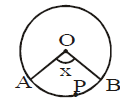2. In fig., if ∠ATO = 40°, find ∠AOB.

[AI-2008]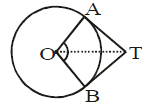3. Find the perimeter of the given figure, where AED is a semi circle and ABCD is a rectangle.

[AI-2008]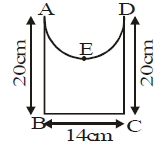4. If the diameter of a semicircular protractor is 14 cm, then find it's perimeter.

[AI-2009]

5. The length of the minute hand of a wall clock is 7 cm. How much area does it sweep in 20 minutes?

[Foreign-2009]

SHORT ANSWER TYPE QUESTIONS

1. In fig., AOBPA is a quadrant of a circle of radius 14 cm. A semicircle with AB as diameter is drawn. Find the area of the shaded region :

[Delhi-2007]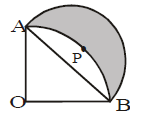2. Four circles are described about the four corners of a square so that each touches two of the others as shown in fig. Find the area of the shaded region, each side of the square is 14 cm. (Take π = 22/7)

[Delhi-2007]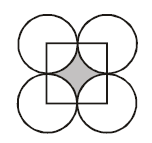3. In the fig., find the perimeter of shaded region where ADC, AEB and BFC are semicircles on diameters AC, AB and BC respectively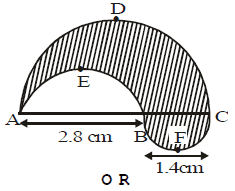Find the area of the shaded region in the fig., where ABCD is a square of side 14 cm.

[Delhi-2008]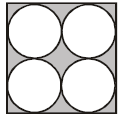4. In fig., ABC is a right-angled triangle, right-angled at A. Semicircles are drawn on AB, AC and BC as diameters. Find the area of the shaded region. A

[AI-2008]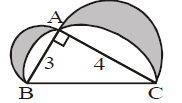5. In the fig., ABC is a quadrant of a circle of radius 14 cm and a semi-circle is drawn with BC as diameter.

Find the area of the shaded region. [Foreign-2008]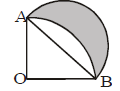6. In fig., PQ = 24 cm, PR = 7 cm and O is the centre of the circle. Find the area of shaded region.

(Take π = 3.14)

[Delhi-2009]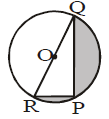7. In figure, the shape of the top of a table in a restaurant is that of a sector of a circle with centre O and ∠BOD = 90°. If BO = OD = 60 cm, find. (i) the area of the top of the table(ii) The perimeter of the table top. (Take π = 3.14)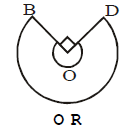In fig., ABCD is a square of side 14 cm and APD and BPC are semicircles. Find the area of shaded region.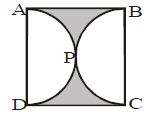(Take π = 22/7)

[Foreign-2009]

8. In fig., AB and CD are two perpendicular diameters of a circle with centre O. If OA = 7 cm. find the area of the shaded region. (Take π = 22/7)

[AI-2010]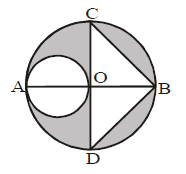LONG ANSWER TYPE QUESTIONS

1. In fig., ABC is a right triangle right angled at A. Find the area of shaded region if AB = 6 cm, BC = 10 cm and O is the centre of the incircle of ΔABC. (Take π = 3.14)

[Delhi-2009]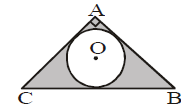2. The area of an equilateral triangle is (49/√3) cm2 . Taking each angular point as centre, circles are drawn with radius equal to half the length of the side of the triangle. Find the area of triangle not included in the circles. (Take √3 = 1.73)

[AI-2009]

VERY SHORT ANSWER TYPE QUESTIONS

1. 100°

2. 100°

3. (7π + 54) cm

4. 36 cm

5. 154/3 cm2

SHORT ANSWER TYPE QUESTIONS

1. 98 cm2

2. 42 cm2

3. 13.2 cm or 42 cm2

4. 6 sq. units

5. 98 cm2

6. 161.3 cm2
7. (i) 9078 cm2
(ii) 402.6 cm or 42 cm2

8. 66.5 cm2

LONG ANSWER TYPE QUESTIONS

1. 11.44 cm2

2. 7.77 cm2

The document Assignment - Areas Related to Circles, Class 10 Mathematics Notes - Class 10 is a part of Class 10 category.
All you need of Class 10 at this link: Class 10
 Use Code STAYHOME200 and get INR 200 additional OFF

Track your progress, build streaks, highlight & save important lessons and more!

,

,

,

,

,

,

,

,

,

,

,

,

,

,

,

,

,

,

,

,

,

,

,

,

;